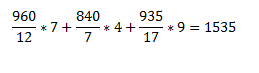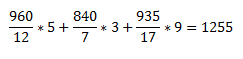# SSC CGL 2018 Practice Test Papers | Quantitative Aptitude (Day-23)

Dear Aspirants, Here we have given the Important SSC CGL Exam 2018 Practice Test Papers. Candidates those who are preparing for SSC CGL 2018 can practice these questions to get more confidence to Crack SSC CGL 2018 Examination.

[WpProQuiz 3026]

Click “Start Quiz” to attend these Questions and view Explanation

1) P, Q, R are employed to do a work for 5890. P and Q together finished 15/31 of work, and Q and R together 10/31 of work. Wage of Q, is–

a) 1190

b) 2500

c) 3570

d) 1140

2) Peter marked the price of watch for Rs. X. But he sold the watch for 2/3 of marked price still his gains 60% discount. What is the ratio of cost price and marked price?

a) 6:11

b) 5:12

c) 2:3

d) 3:2

3) The income of Asmitha and Bindu are in the ratio 1 : 2. They spend in the ratio 3: 5 and save in the ratio 1 : 4. If the total monthly savings of both Asmitha and Binduare 5000, the monthly income of Bindu is

a) 20000

b) 25000

c) 11100

d) 14000

4) The ratio of the sum of two numbers and their difference is 7 : 1. The ratio of the greater Number to the smaller number is

a) 3:4

b) 4:3

c) 2:1

d) 1:2

5) The average of three numbers A, B and C is 86. The first number is thrice the second and the difference between second number and first number is equal to the third number.  Find the value of the first number.

a) 135

b) 129

c) 200

d) 178

6) If tanθ.tan 2θ= 1, then the value of sin2 2θ+tan2 2θ is equal to __

a) 4 /3

b) 3 /10

c) 3 3/4

d) 3

Direction (7- 8): Refer the below diagram and answer the following questions.

In a class of 50 students, the number of students passed in Maths is represented as Circle and the number of students passed in English is represented as Rectangle and the number of students passed in Economics is represented as triangle.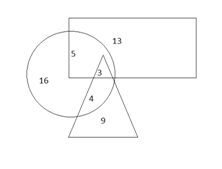7) If the number of students passed in English alone increased by 100% then what is the number of students passed in English?

a) 34

b) 24

c) 26

d) 30

8) What is the ratio of number of students passed in both Maths and English and the number of students passed in Maths alone?

a) 2:1

b) 1:2

c) 1:1

d) 3:1

Direction: (9 –10) Refer the below table and answer the following questions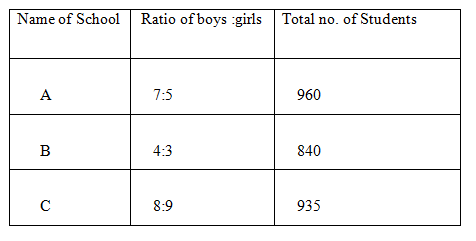9) What is the sum of number of boys in schools A and B and number of girls in School C?

a) 1335

b) 1535

c) 1355

d) 1435

10) Find the total number of girls.

a) 1300

b) 1175

c) 1255

d) 1322x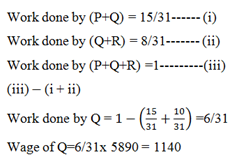Let the marked price of watch be Rs. 300

Selling price = 2/3×300 = 200

Profit = 60%

Cost price = 100×200/160 = 125

Ratio of CP: MP = 125: 300

=5:12

Income of Asmitha = x

Income of Bindu = 2x

Expenditure of Asmitha = 3 y

Expenditure of Bindu = 5y

Savings of Asmitha = z

Savings of Bindu = 4z

z+4z=5000

5z=5000

Z=1000

Income – expenditure= Savings

For Asmitha,

X-3y=1000—— (i)

For Bindu,

2x-5y=4000——- (ii)

Solve (i) and (ii),

X=7000

Y=2000

Monthly salary of Bindu= 2x=2×7000=14000

Let the two numbers be x and y respectively.

(x+y): (x-y) = 7:1

x:y = 4:3

Sum of three numbers= 86×3= 258

Ratio of A, B and C= 3:1:2

Sum of A, B, C=6x

6x=258

X=43

A= 3×43=129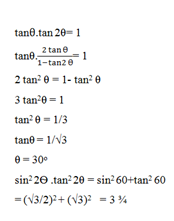13+100/100*13+5+3=34

5+3:16=1:2